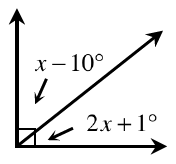Home > GB8I > Chapter cc41 > Lesson cc41.2.3 > Problem1-62

1-62.Rosalinda examined the angles at right and wrote the equation below. Homework Help ✎

$(2x + 1°) + (x – 10°) = 90°$

1. Does her equation make sense? If so, explain why her equation must be true. If it is not correct, determine what is incorrect and rewrite the equation.

• Is a right angle (as illustrated above), equal to 90°?

Yes. This means Rosalinda's equation makes sense, because the two angles make up a 90° angle.

1. If you have not already done so, solve her equation, clearly showing all your steps. What are the measures of the two angles?

• Refer to the Math Notes box in Lesson 1.1.4 if you need help remembering how to solve a linear equation.

1. Verify that your answer is correct.

• After solving for x, substitute your answer into the original equation to verify it is correct.